# Calculating the Interest Rate (i)

If we know the present value (PV), the future value (FV), and the number of time periods of compound interest (n), future value factors will allow us to calculate the unknown interest rate (i). Calculations #9 through #12 illustrate how to determine the interest rate (i).

### Calculation #9

A single investment of \$500 is made today and will remain invested for 5 years. At the end of the 5th year, the future value will be \$669. Assuming that the interest is compounded annually, calculate the annual interest rate earned on this investment.

The following timeline plots the variables that are known and unknown: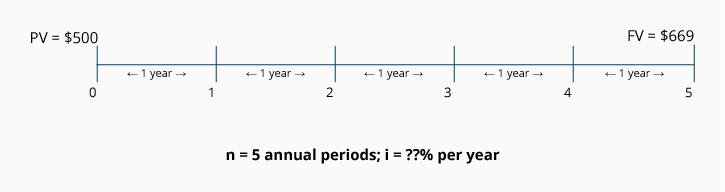Calculation using the FV of 1 Table: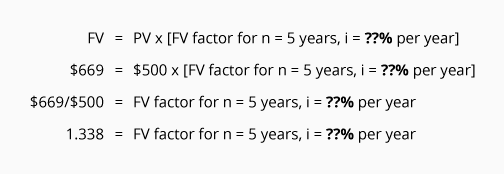To finish solving the equation, we search only the "n = 5" row of the FV of 1 Table for the FV factor that is closest to 1.338. In this case, there is a factor of 1.338, and it is located in the column with the heading i = 6%.

Since the time periods are one-year periods, the interest rate is 6% per year compounded annually.

### Calculation #10

A basket of goods has a cost today of \$100. Assume that the cost is estimated to increase to \$180 at the end of 6 years. What is the annual rate of increase if the cost increases are compounded semiannually?

The following timeline plots the variables that are known and unknown: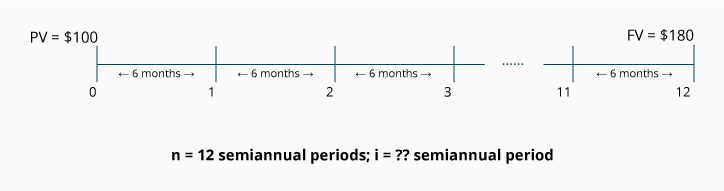Because the rate of increase (the "interest") is compounded semiannually, we convert the 6 years to 12 semiannual time periods.

Calculation using the FV of 1 Table: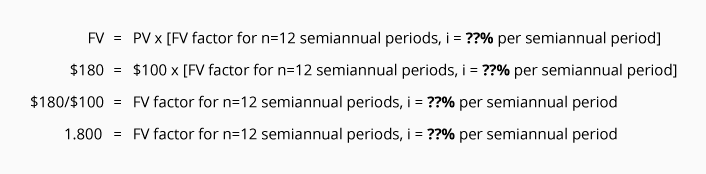To finish solving the equation, we search only the row "n = 12" of the FV of 1 Table for the FV factor that is closest to 1.800. In this case, there is a factor of 1.796 located in the column where i = 5%.

Since (n) represents semiannual time periods, the rate of 5% is the semiannual rate, or the rate for a six-month period. To convert the semiannual rate to an annual rate, we multiply 5% x 2, the number of semiannual periods in a year. This means that the rate of increase for the basket of goods is 10% per year compounded semiannually.

### Calculation #11

Assume you invest \$100 today and intend to keep it invested for 6 years. You are told that at the end of the 6th year, the future value of your account will be \$161. Assuming that the interest is compounded quarterly, compute the annual interest rate you are earning on this investment.

The following timeline plots the variables that are known and unknown: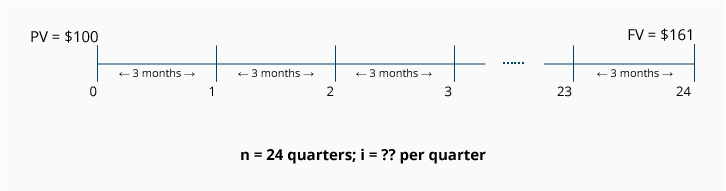Because the interest is compounded quarterly, we convert the 6 years to 24 quarterly time periods. In other words, we will refer to n = 24 when using the FV of 1 Table.

Calculation using the FV of 1 Table: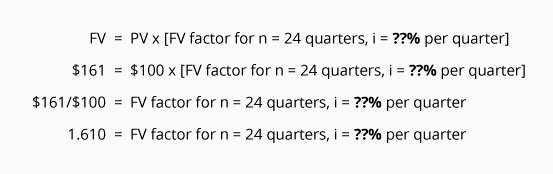To finish solving the equation, we search only the row where n = 24 in the FV of 1 Table for the future value factor. We look for the FV factor that is closest to 1.610. In this case, a factor of 1.608 is located in the column where i = 2%.

Since 2% is the interest rate per quarter, we multiply the quarterly rate of 2% x 4, the number of quarterly periods in a year. Hence the investment is earning an interest rate of 8% per year compounded quarterly.

### Calculation #12

Aaron has a sum of \$500 and he needs for it to grow to a future value of \$634 by the end of one year. Assuming that the interest rate is compounded monthly, what interest rate does Aaron need for his investment?

The following timeline plots the variables that are known and unknown: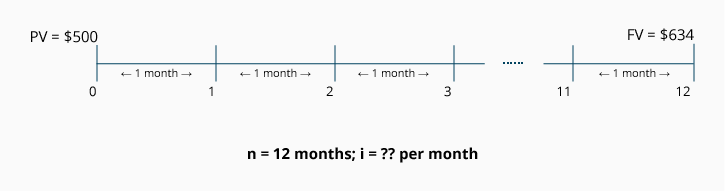Because the interest is compounded monthly, we convert the 1 year time period to n = 12 monthly time periods.

Calculation using the FV of 1 Table: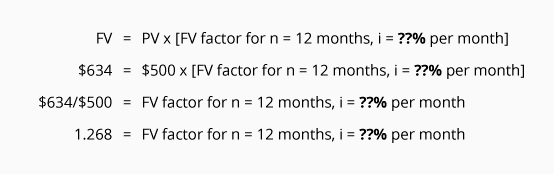To finish solving the equation, we search only the row where n = 12 in the FV of 1 Table for the FV factor that is closest to 1.268. In this case, the factor of 1.268 is located in the column where i = 2%.

Since i = 2% is the monthly rate, we multiply 2% x 12, the number of monthly periods in a year in order to determine the annual rate. In this case, Aaron needs to find an interest rate of 24% per year compounded monthly in order to reach his future value goal of \$634 in one year.